Relativity, Theory of

Also found in: Dictionary.
Related to Relativity, Theory of: special relativity theory
The following article is from The Great Soviet Encyclopedia (1979). It might be outdated or ideologically biased.

Relativity, Theory of

a physical theory dealing with the space-time properties of physical processes. The laws established by the theory of relativity are common to all physical processes and therefore are often referred to simply as properties of space-time. As established by A. Einstein, these properties depend on the gravitational fields acting in a given region of space-time. The properties of space-time in the presence of gravitational fields are investigated in the general theory of relativity, which is also a theory of gravitation. The special theory of relativity considers the properties of space-time in an approximation in which the effects of gravitation can be ignored. The special theory of relativity is logically a special case of the general theory of relativity; hence its name. The development of the theory historically took place in the reverse order: the special theory of relativity was formulated by Einstein in 1905, and the final statement of the general theory of relativity was given by him in 1916. The special theory of relativity, also called simply the theory of relativity or Einstein’s theory of relativity, is the subject of the present article, which concludes with an account of the theory’s origins (see below: History of the special theory of relativity).

Basic features of the theory of relativity. The phenomena described by the theory of relativity and called relativistic appear when bodies move at speeds close to the speed of light in a vacuum, c = (2.997924562 ± 0.000000011) × 1010 cm/sec. At such speeds, which are said to be relativistic, the dependence of the energy E of a body on its speed v is no longer given by the equation of classical mechanics for kinetic energy Ekin = mv2/2 but by the relativistic formula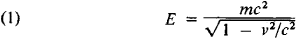The mass m in this formula is also called the rest mass in the theory of relativity. It can be seen from (1) that the energy of a body tends toward infinity as v tends toward c, and therefore if the rest mass is nonzero the speed of the body is always less than c. When E ≫ mc2, however, the speed can become arbitrarily close to c, an effect that is directly observed in proton and electron accelerators. When energies much greater than mc2 are imparted to particles in such accelerators, the particles move with a speed practically equal to c. Particles whose rest mass is equal to zero—photons (light quanta) and neutrinos—always move with the speed of light. The speed c is the limiting speed of transmission of any interactions or signals from one point in space to another.

The existence of a limiting speed necessitates a fundamental change ir. conventional concepts of space-time, which are based on everyday experience. Let us imagine that in a railroad car moving with a speed v relative to the roadbed a light signal is sent in the direction of motion of the car. For an observer in the car the speed of the signal is equal to c. If the lengths and times measured by any observer were identical, then the law of addition of velocities of classical mechanics would be obeyed and for an observer standing beside the roadbed the speed of the signal would be equal to c + v; that is, it would be greater than the limiting speed. The contradiction is resolved by the fact that in reality, from the point of view of an observer relative to whom a physical system moves with a speed v, all processes in the system are slowed down by the factor ofa phenomenon called time dilatation. The longitudinal dimensions, or dimensions in the direction of motion, of bodies are shortened by the same factor. In addition, events that are simultaneous for one observer turn out to be nonsimultaneous for another observer who is in motion relative to the first observer, a phenomenon called relativity of simultaneity. Consideration of these effects leads to a law of addition of velocities where the limiting speed turns out to be identical for all observers.

The time dilatation phenomenon, which is characteristic of the theory of relativity, can assume a vast scale. Decaying (unstable) particles moving at a speed close to that of light are produced in accelerator experiments and in cosmic rays. As a result of time dilatation, the particles’ decay times and consequently the distances traversed by the particles from creation to decay are, from an earthbound observer’s point of view, thousands or tens of thousands of times greater than the distances the particles would travel if the time dilatation effect were absent.

It follows from the relativistic energy formula that for small speeds (v ≪ c) the energy of a body is equal toThe second term on the right is the usual expression for the kinetic energy. The first term indicates that a body at rest has an energy content E0 = mc2, which is called the rest energy. The formula E0 = mc2 expresses the principle of the equivalence of mass and energy, or the Einstein mass-energy relation.

In nuclear reactions and in the transformations of elementary particles, a large part of the rest energy can become the kinetic energy of particles. Thus, the transformation of four protons into a helium nucleus is the source of the energy radiated by the sun. Since the mass of the helium nucleus is 4.8 × 10-26 g less than that of the four protons, during each such transformation there is released 4.3 × 10-5 erg of kinetic energy, which is carried away by radiation. The sun loses 4 ×106 tons of its mass per second through radiation.

The theory of relativity has been confirmed by a broad range of facts and underlies all modern theories that deal with phenomena at relativistic speeds. A consistent theory of electromagnetic phenomena, particularly optical phenomena, that are described by classical electrodynamics as exemplified in Maxwell’s equations is possible only on the basis of the theory of relativity. The theory also underlies quantum electrodynamics and the theories of the strong and weak interactions of elementary particles. The laws governing the motion of bodies at relativistic speeds are considered in relativistic mechanics, which for speeds v ≪ c becomes classical Newtonian mechanics. The quantum laws of the motion of relativistic microparticles are dealt with in relativistic quantum mechanics and quantum field theory.

The principle of relativity and other in variance principles. The relativity principle lies at the foundation of the theory of relativity. According to the principle, for an observer moving with a physical system that has been placed in a state of free uniform and linear motion relative to a system arbitrarily defined to be at rest, all processes obey the same laws as in the rest system. The moving system is said to be derived from the rest system by a transformation of motion, and the principle of relativity is said to express the invariance, or independence, of the laws of nature with respect to transformations of motion.

The validity of the relativity principle means that the difference between a state of rest and a state of uniform linear motion has no physical significance. If a physical system B moves uniformly and linearly with a velocity V relative to a system A, then we have an equal right to say that A moves relative to B with the velocity —V. The term “relativity principle” is used because all relative motions of the bodies of a system of moving bodies remain unchanged under a transformation of motion.

Besides the principle of relativity, there are known from experiment other principles of invariance, or symmetry, of the laws of nature. Any physical process occurs in precisely the same way:

(a) if it is carried out at any other point of space; this symmetry expresses the equivalence of all points in space, or the homogeneity of space;

(b) if a system if which the process occurs is rotated by an arbitrary angle; this symmetry expresses the equivalence of all directions in space, or the isotrophy of space;

(c) if the process is repeated after some time interval; this symmetry expresses the homogeneity of time.

Thus, invariance of the laws of nature holds with respect to four types of transformations: (1) translation in space, (2) rotation in space, (3) translation in time, and (4) transformation of motion. Symmetries (l)-(4) are satisfied exactly only in a system isolated from external influences; that is, only if the effect of external factors on the system can be ignored. For real systems the symmetries are only approximately valid.

The study of the properties of transformations (1) and (2) is the subject of three-dimensional Euclidean geometry understood as a physical theory describing the spatial properties of physical objects. Translation here should be interpreted as the transformation of a parallel translation.

For bodies moving at speeds v comparable with c, a close relation and mathematical analogy is observed between transformations (1) and (3) and transformations (2) and (4). There thus are grounds for speaking of the theory of relativity, in which transformations (l)-(4) should be considered jointly, as a geometry of space-time. The theory of relativity is concerned with the properties of transformations (l)-(4) and with the consequences of the corresponding invariance principles. Mathematically, the theory of relativity is a generalization of Euclidean geometry— the geometry of four-dimensional Minkowski space.

The principle of relativity was known and was valid in classical mechanics, but the properties of transformations of motion are different for v ≪ c and v ~ c. When v ≪ c, relativistic effects vanish and the transformations of motion become the invariance transformations that are valid for classical mechanics (Galilean transformations). The relativistic principle of relativity, usually called Einstein’s relativity principle, is therefore to be distinguished from the Galilean nonrelativistic principle of relativity.

The fundamental concept of the theory of relativity is the point event, that is, something that occurs at a given point in space at a given moment in time, for example, a light flash or the decay of an elementary particle. This concept is an abstraction —real events always have some extent in space and time and can be considered as point events only approximately. Any physical process is a sequence of events (C)—C1, C2, …, Cn,. The validity of symmetries (l)-(4) means that in addition to the sequence (C) the laws of nature permit the existence of an infinite number of other sequences (C*) that are derived from (C) by appropriate transformations. These sequences differ in the position of the events in space and time, but their internal structure is identical with that of (C). For example, symmetry (4) can be illustrated by a process (C) that occurs in an aircraft standing on the ground, while the process (C*) is the same process occurring in an aircraft flying at a constant speed relative to the earth; different sequences (C*) correspond to different speeds and directions of motion.

Transformations that convert one sequence of events to another are said to be active; passive transformations link the coordinates of a given event in two frames of reference (see below). A set of active transformations must have certain properties. First, the successive application of any two transformations must represent one of the possible transformations, for example, the transition from a first system to a second system, and then from the second system to a third system, is equivalent to the transition from the first system to the third system. Moreover, for every transformation there must exist an inverse transformation such that successive application of the two transformations yields an identity transformation that is one of the possible transformations of the system. This means that the set of transformations (l)-(4) must constitute a group in the mathematical sense. This group is called a Poincaré group, a name proposed by E. Wigner. The transformations of a Poincaré group are universal in character; they act in the same way on events of any type. This fact makes it possible to assume that they describe properties of space-time rather than of specific processes. The properties of Poincaré transformations can be described in different ways just as the properties of motion in three-dimensional space can be described in different ways. The simplest description uses inertial frames of reference and clocks associated with the frames. The role of inertial frames of reference in the theory of relativity is the same as that of rectangular Cartesian coordinates in Euclidean geometry.

Inertial frames of reference. The same degree of accuracy found when the properties of a given region of space-time are described by the special theory of relativity can be obtained with the introduction of inertial frames of reference, in which the space-time laws of the theory of relativity assume a particularly simple form. In this case, a frame of reference can be understood as a rigid system of solid bodies (or an imaginary extension thereof) relative to which the positions of events and the trajectories of bodies and light rays are defined. Any frame of reference moving uniformly, linearly, and without rotation relative to an inertial frame of reference will also be inertial; a frame of reference that rotates or moves with acceleration will no longer be an inertial frame of reference. Consequently, inertial frames of reference form a special class of frames of reference. The law of inertia is valid in an inertial frame of reference; that is, a free particle, or a particle not influenced by other bodies, moves linearly and, if the clocks are synchronized properly (see below), uniformly in an inertial frame of reference. The requirement that the law of inertia be satisfied can be adopted as the definition of inertial frames of reference. Newton’s first law can be regarded as a statement that such frames of reference exist. All inertial frames of reference are equivalent, and this equivalence is a direct expression of the principle of relativity.

The degree to which a frame of reference is inertial depends on the properties of the gravitational fields acting in the region of space-time being considered. The quantitative criteria for the applicability of the special theory of relativity and for the inertial nature of a frame of reference are considered in the general theory of relativity.

Noninertial frames of reference can also be used in a region of space-time in which the special theory of relativity is valid, just as curvilinear coordinates can be used in Euclidean geometry. The description of the properties of space-time, however, proves to be more complicated for noninertial frames.

For a given inertial frame of reference it is necessary to define the method used to measure time and position coordinates. In an inertial frame of reference, three-dimensional spatial geometry is Euclidean if straight lines are defined, for example, as the trajectories of light rays and distances are measured by rigid scales. The rectangular Cartesian coordinates x, y, and z can therefore be introduced in the given inertial frame of reference. To determine the time t of an event, a clock at rest in the given inertial frame of reference can be assumed to be located at the point where the event occurred. If events occur at different points A and B, then in order to compare the events’ times the clocks at A and B must be synchronized—that is, a definition must be given of what it means to say that the clocks at A and B show the same time. The usual definition involves sending a signal from A to B at time tA according to the clock at A and then sending the same signal from B to A at the moment the signal arrives at B. If the signal arrives at A at time tA′, then the signal is assumed to have arrived at B at the time tB = (tA + tA′)/2, and the clock at B is set correspondingly. Under this definition, the travel times for the signal from A to B and from B to A are identical, being (tA′ — tA)/2. Light flashes, sound signals (if the medium in which they are propagated is at rest relative to the given frame of reference), shots from two identical guns mounted at A and B, and so on can serve as the signal, as long as the conditions for transmission of the signal from A to B and from B to A are the same.

This definition of time is appropriate because no inertial frame of reference contains a physically preferred direction. The procedure described above for synchronizing clocks is symmetric with respect to A and B and therefore does not introduce anisotrophy into the method of description. The lack of a preferred direction is reflected in the fact that synchronization by any signal yields the same result. The same result is obtained also if the clock at A is moved at a low speed, v ≪ c, to B. In practical measurements of time and position coordinates, there are numerous indirect methods that can be used, as long as they give the same result as the procedures described above. In another inertial frame of reference, position coordinates and time are measured by means of the same scales and clocks, which are synchronized in the same manner. It is not obvious in advance that the times defined in this manner in two different inertial frames of reference will be the same, and in fact they turn out to be different. After synchronization is carried out, the speeds of particles and signals in the given inertial frame of reference, in particular the rate of propagation of light signals, can be measured. The speed of light in any inertial frame of reference is always equal to c.

Lorentz transformations. The active transformations considered above are directly related to passive transformations that describe the connection between a given event’s position and time coordinates in two different inertial frames of reference. By virtue of the principle of relativity, it makes no difference whether a body is given a velocity V relative to a particular inertial frame of reference L or whether a frame of reference L’ moving with a velocity V relative to L is adopted—the law of transformation of position and time coordinates must be the same.

Because of the validity of symmetries (l)-(4), any transformation that links an event’s position and time coordinates x, y, z. and t in the inertial frame L and the event’s coordinates x’, y’, z’, and t’ in the inertial frame of reference L’ must be linear. The form of these transformations can be derived from symmetries (l)-(4) and from the requirement that the transformations constitute a group. Let us suppose the frame of reference L’ moves relative to L with a velocity V. Let the coordinate axes and origins in L and L’ be chosen so that the x- and x’-axes coincide and are directed along V, the y- and y’-axes are parallel, the z- and z’-axes are parallel, the origins O and O’ coincide when t = 0, and the clock in L’ is set so that when t = 0 the clock at O’ shows the time t’ = 0. Under these conditions, the coordinate transformations have the form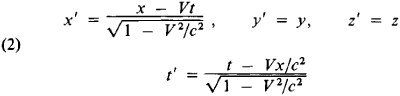where c is an arbitrary constant that indicates the maximum rate of motion and is equal to the speed of light in a vacuum. This constant can be determined from any effect of the theory of relativity, for example, the slowing-down of the decay time of a fast π-meson. The validity of the kinematics and dynamics based on transformations (2) has been confirmed by a great number of experimental facts.

Together with the transformations of rotation about the origin of coordinates, the Lorentz transformations (2) form a Lorentz group. If to the Lorentz group we add the translations in time t’ = t + a and in space x’ = x + b, where a and b are arbitrary constants with the dimensions of time and length, respectively, we obtain a Poincaré group.

It follows from the principle of relativity that physical laws must have an identical form in all inertial frames of reference; consequently, they must preserve their form under Lorentz transformations. This requirement is called the principle or postulate of the relativistic invariance, Lorentz invariance, or Lorentz covariance, of the laws of nature.

The relativistic law of addition of velocities follows from the Lorentz transformations. If a particle or signal moves in L along the x-axis at a speed v, then, at time t, x = vt and the particle’s speed v’ = x’/t’ measured in the L’ frame is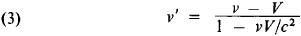This formula expresses a fundamental feature of relativistic kinematics—the speed of light is independent of the motion of the source. Indeed, if the speed of the light emitted by a source at rest in some inertial frame of reference L is c (v = c), then it follows from the law of addition of velocities (3) that the speed of light v’ measured in the inertial frame of reference L’ is also equal to c. Consequently, since the direction of the x-axis is arbitrary, the speed of light is independent of the motion of the source. This property of the speed of light unambiguously determines the form of the Lorentz transformations: the transformations can be derived by postulating the independence of the speed of light from the motion of the source, the homogeneity of space and time, and the isotropy of space.

The speed of light plays a special role in the theory of relativity because it is the limiting speed for the propagation of signals and the motion of particles. As a particle’s energy tends toward infinity or its mass tends toward zero, the particle’s speed approaches the speed of light. It has been experimentally established that a photon’s rest mass m γ < 4 X 10-21me, where me is the mass of the electron. Were m γ to be found to be nonzero, the speed of light would be less than the limiting speed. In order for the limiting speed to exist in general, it must be independent of the motion of the particle source.

It is easy to obtain from the Lorentz transformations the basic effects of the theory of relativity: the relativity of simultaneity, time dilatation, and the contraction of the longitudinal dimensions of moving bodies. Indeed, if an event 1 and an event 2 are simultaneous in an inertial frame of reference L (t1= t2) and occur at different points x1 and x2, they are found to be non-simultaneous in another inertial frame of reference L’: t1′ —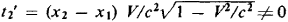Furthermore, when a clock at rest in L at the point x = 0 shows the time t, then the time t’ on a clock in L’ that is spatially coincident with the clock in L at this moment of time is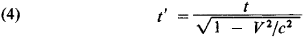Thus,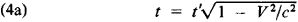that is, from the point of view of an observer in L’ the clock in L is slow. By virtue of the principle of relativity, it follows that from the point of view of an observer in L’ all processes in L are slowed by an equal factor.

It also is easy to show that the dimensions l of all bodies at rest in L turn out to be shortened by the factor of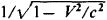in the direction of V when they are measured in L ′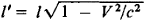In particular, the longitudinal diameter of a sphere moving at the speed v relative to L’ will be, when measured in shorter than the transverse diameter by the factor of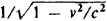. It should be noted that this contradiction would not be observed in an instantaneous photograph of the sphere. Because the light signals arriving from different points of the sphere experience different delays, the apparent shape of the sphere would remain unchanged.

For inertial frames of reference, the space-time effects determined by the Lorentz transformations are relative: from the point of view of an observer in L, all processes are slowed and all longitudinal scales are shortened in L’. This statement, however, does not hold if at least one of the frames of reference is noninertial. If, for example, clock 1 moves, relative to L, from A to B with a speed v and then from B to A with a speed — v, it will be slow in comparison with clock 2, which is at rest at A, by the factor of. This result can be observed by direct comparison; thus the effect is absolute. The effect must hold for any process. For example, a twin who makes a trip at a speed v will return younger by the factor of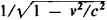than his brother who remains stationary in the inertial trame of reference. This phenomenon, which is called the twin paradox, actually does not involve a paradox at all. The frame of reference associated with clock 1 is not inertial: when clock 1 turns at B, it undergoes an acceleration relative to the inertial system. Clocks 1 and 2 are therefore nonequivalent.

For small v the Lorentz transformations become the Galilean transformations x’ = x — vt, y’ = y, z’ = z, and t’ = t. These transformations describe the relation between the pictures seen by different observers that is known from everyday experience, where the dimensions of objects and the duration of processes are identical for all observers.

The Poincaré transformations leave invariant the quantity called the interval SAB between the events A and B. The interval is defined by the relation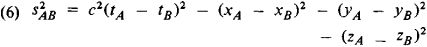Mathematically, the invariance of s is analogous to the invari-ance of distance under transformations of motion in Euclidean geometry. The quantities ct, x, y, and z may be considered as the four coordinates of an event in four-dimensional Minkowski space: x0 = ct, x1 = x, x2 = y, and x3 = z, which are the components of a four-dimensional vector.

If the imaginary coordinate x4 = ix0 = ict is introduced instead of x0, then any Poincaré transformation can be written in a form analogous to the formula that describes rotations and translations in three-dimensional space.

Because the squares of the differences of the time and space coordinates occur in (6) with different signs, the sign of s2 can vary. The geometry of this space differs from Euclidean geometry and is referred to as quasi-Euclidean. In this geometry the intervals are of three types: s2 < 0, s2 > 0, and s2 = 0. Intervals of the first and second types are called timelike and spacelike, respectively. If s2 ≥ 0, then the sign of tAtB is independent of the frame of reference. This fact is closely connected with the causality principle. Indeed, if s2 ≥ 0 and, for determinacy, tA< tB, then events A and B can be connected by a signal propagated at a speed v≤c; that is, A can be the cause of B. Conventional ideas of causality then require that in any frame of reference event B follow event A. The invariance of the condition s2 = 0 is a direct expression of the invariance of the speed of light. If s2 < 0, then the sign of tAtB can differ in different inertial frames of reference. This, however, is not inconsistent with causality, since such events cannot be connected by any interaction.

If s2 < 0, there exists a frame of reference such that the events A and B are simultaneous; in this system s2 = - l 2, where l is conventional distance. When s2 > 0, there exists a frame of reference in which events A and B occur at the same point.

In classical physics the requirement that physical laws be invariant under Lorentz transformations means that physical quantities must be transformed as scalars, vectors, or tensors in Minkowski space. The rules for calculations involving such quantities are given by the tensor calculus. Use of the tensor calculus makes it possible to write physical laws in such a form that the Lorentz invariance of the laws becomes directly apparent.

Laws of conservation in the theory of relativity and relativistic mechanics. In the theory of relativity, as in classical mechanics, the momentum p and energy E are conserved for a closed physical system. The three-dimensional vector of momentum, together with energy, forms the four-dimensional momentum-energy vector with the components E/c and p, written (E/c, p). The quantity

E2 - (cp)2 = m2c4

where m is the rest mass of the particle, remains invariant under Lorentz transformations. It follows from the requirements of Lorentz invariance that the dependence of energy and momentum on velocity has the formThe relation between the energy and momentum of a particle is given by the equation p = Ev/c2. This equation also holds for a particle with zero rest mass; then v = c and p = E/c. Photons (γ), electron neutrinos, and muon neutrinos apparently are such particles. It can be seen from (8) that the momentum and energy of a particle with m 0 tend toward infinity as vc.

The possibility of the existence of tachyons, or particles that move at a speed greater than that of light, has been discussed. The existence of tachyons is not formally inconsistent with Lorentz invariance, but it leads to serious complications in satisfying the causality requirement.

The rest mass m is not a conserved quantity. In particular, in the processes of decays and transformations of elementary particles, the sum of the energies and momenta of the particles is conserved, but the sum of the rest masses changes. Thus, in the process of the annihilation of a positron and an electron, e+ + e- → 2 γ, the sum of the rest masses changes by 2me.

A frame of reference in which a body is at rest is said to be intrinsic. As has already been mentioned, the energy of the body in such a frame is E0= mc2. If a body, while remaining at rest, changes state by receiving energy in the form of radiation or heat, the relativistic law of conservation of energy requires that the relation between the energy Δ E acquired by the body and the increase Δ m in the body’s rest mass be given by the equation ΔE = Δmc2. It follows from this relation, which is known as Einstein’s principle of the equivalence of mass and energy, that the quantity E0= mc2 defines the maximum energy that can be “extracted” from a given body in a reference frame in which the body is at rest.

The kinetic energy of a moving body is defined as the quantity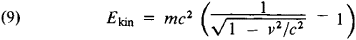When vc, equation (9) becomes the nonrelativistic expression Ekin= mv2/2. Here the momentum is equal to p = mv. It follows from the definition of Ekin that for any process in an isolated system the equation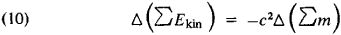is satisfied. According to this equation, the increase in kinetic energy is proportional to the decrease in the sum of the rest masses. The equation is used extensively in nuclear physics; it permits the prediction of the energy release in a nuclear reaction if the rest masses of the particles involved are known. The possibility of processes in which the conversion of rest energy into kinetic energy of particles occurs is limited by other laws of conservation. For example, the law of conservation of baryon charge forbids the conversion of a proton into a positron and a γ = quantum.

The mass defined as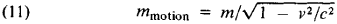is sometimes introduced. Here the relation between momentum and velocity has the same form as in Newtonian mechanics: P = mmotionv. The mass defined in this manner differs from the energy of the body only by the factor 1/c2. In fact, in theoretical physics the units of measurement are often selected so that c = 1 and consequently E = mmotion.

The basic equations of relativistic mechanics have the same form as Newton’s second law and the equation of energy, but expressions (8) are used instead of the nonrelativistic expressions for energy and momentum: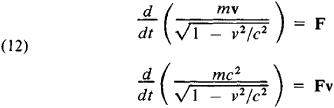where F is a force acting on the body. For a charged particle moving in an electromagnetic field, F is the Lorentz force.

The theory of relativity and experiment. The hypotheses concerning point events, the validity of the relativity principle, the homogeneity of time, and the homogeneity and isotropy of space inevitably lead to the theory of relativity. In addition, the abstract possibility that the limiting speed c = ∞ has been experimentally precluded. The value of c has been proved with extreme precision (see below) to be the speed of light in a vacuum.

What are the limits of applicability of the theory of relativity? The deviations from the space-time geometry of the theory of relativity that are connected with gravitation are observable and are calculated in the general theory of relativity. No other limitations on the applicability of the theory of relativity have been found thus far, although suggestions have often been advanced that at very small distances, for example, < 10-17 cm, the concept of point event and consequently the theory of relativity may prove to be inapplicable. In this connection attempts have been made at space-time quantization.

The hypothesis of Lorentz invariance and of the point nature of events, that is, the local nature of interactions, underlies all present-day theories in which relativity plays an essential role.

The validity of the quantum electrodynamics of electrons and muons, and consequently the validity of the theory of relativity, has been established for distances as small as 10-15 cm. The agreement of quantum electrodynamics with experiment has been established for energies of the order of the masses of these particles to within one part in 105; the mechanics of the theory of relativity should be valid with the same order of accuracy.

The relativistic laws of conservation are used in research on the transformations of elementary particles induced by strong, weak, and electromagnetic interactions. The absence of contradictions attests to the validity of the laws. All that is known about the interactions is in agreement with the notion of their Lorentz invariance.

The hypothesis that faster-than-light signals are impossible follows from the theory of relativity and is the basis for the dispersion methods widely used in the theory of strong interactions. The success of these methods is evidence of the validity of the basic concepts of the theory of relativity.

One of the most spectacular confirmations of the validity of relativistic invariance was Dirac’s prediction, on the basis of that invariance, of the existence of antiparticles and the subsequent discovery of such particles.

The requirement of the Lorentz invariance of interactions leads, in very general hypotheses, to the CPT theorem, which defines the relation between the properties of particles and antiparticles. This relation has been experimentally confirmed for all known interactions.

Many experiments have been performed to verify directly the main features of the kinematics of the theory of relativity. The most accurate test of whether the speed of light is independent of the motion of the source was carried out in 1964 at the European Center for Nuclear Research (CERN) in Switzerland. The experiments made use of γ -quanta from the decay of the π °-meson. For a speed of the π0 -meson of v = 0.9997c, the speed of the γ -quantum agreed with c to within one part in 104. Relativistic time dilatation has been measured in a wide range of speeds by means of the transverse Doppler effect and directly from the decays of elementary particles with an accuaracy of 1–5 percent. The formula mmotion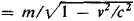has also been verified many times; the highest accuracy, one part in 5 × 104, was attained by V. Meyer and his colleagues in 1963.

History of the special theory of relativity. Although the theory of relativity is simple in a logical sense, the path that led to it was quite complicated. The validity of the relativity principle for mechanical phenomena and the principle’s connection with the phenomenon of inertia were grasped after the appearance of the theory of N. Copernicus. The lack of apparent manifestations of the earth’s motion inevitably led to the conclusion that the overall motion of a system does not affect the mechanical phenomena occurring in the system. As early as the 16th century this idea was illustrated by a description of experiments on a moving ship. The classical exposition of the principle of relativity was given in 1632 by Galileo: “Have the ship proceed at any speed you like so long as the motion is uniform and not fluctuating this way and that. You will discover not the least change in all the efforts named, nor could you tell from any of them whether the ship was moving or standing still” (Galileo, Dialog o dvukh glavneishikh sistemakh mira: ptolomeevoi i kopernikovoi, Moscow-Leningrad, 1948, p. 147). The principle of relativity was used extensively by C. Huygens in solving problems of mechanics.

A complete system of laws of motion for any mechanical system was given by I. Newton in Principia (1687). After establishing that the laws of mechanics cannot be valid in all frames of reference, Newton introduced the concepts of absolute space and absolute time. For him these concepts were essentially the reference frame and the time variable t for which the laws of motion were satisfied. The measurement of time was a simple problem in Newtonian mechanics, since any uniformly moving clock was suitable for measuring t. The question of absolute space was more complicated. The principle of relativity was satisfied in Newtonian mechanics. In Newton’s formulation, “the relative motions of bodies enclosed in a space are identical regardless of whether the space is at rest or is moving uniformly, linearly, and without rotation” (“Matematicheskie nachala natural’noi filosofii,” in A. I. Krylov, Sobr. trudov, vol. 7, 1936, p. 49). It was therefore impossible to distinguish a frame of reference at rest in absolute space from a uniformly moving frame. A transition from one inertial frame of reference to another was described in Newtonian mechanics by the transformations x′ = x – vt and t’ = t, which are now called Galilean transformations. The form of the transformations seemed self-evident, since there was no doubt that the lengths of objects are identical in all frames of reference and that time is unique. This confidence was bolstered by the invariance of Newton’s laws with respect to Galilean transformations. The invalidity of the principle of relativity for optical phenomena seemed just as indisputable. As early as the 17th century, extensive use was made of the concept of a medium filling space—the ether. The transmission of light disturbances was among the many functions ascribed to the ether. The optics of T. Young and A. Fresnel, which was worked out in the early 19th century, regarded the speed of light to be constant relative to the ether and to be independent of the motion of the source. This conception implied a violation of the principle of relativity, since for an observer moving in the ether at a speed v the speed of oncoming light should be equal to c + v —an effect referred to as the ether wind. The ether wind was supposed to arise, in particular, as a result of the earth’s orbital motion at a speed of 30 km/sec. The search for the ether wind was complicated, however, by the circumstance that even according to Fresnel’s theory, effects of the order of v/c (∼ 10-4 for the earth’s orbital motion) should be absent in a broad class of experiments.

The existence of the ether became one of the central problems of physics after J. Maxwell’s construction of an electromagnetic field theory in which the ether is the carrier of electric and magnetic fields, in addition to light waves. Attempts to detect the ether wind were made by A. Michelson in 1881 and by Michel-son together with E. Morley in 1887, who sought an effect of the order of v2/c2; the results were negative. The problem arose of reconciling Michelson’s experiment with optics and electrodynamics, which were founded on the concept of the ether. Explanations involving the hypothesis that the ether is completely entrained by moving bodies seemed the most obvious. Optical and electromagnetic theories that made use of this hypothesis were discussed by G. G. Stokes and H. Hertz, but they either turned out to be internally contradictory or did not describe the entire body of experimental facts. The electrodynamics of H. Lo-rentz, which was based on the concept of the fixed ether and which at first glance seemed incompatible with the principle of relativity, was the most successful. In 1892, Lorentz found, as the British physicist G. Fitzgerald had in 1889, that the negative result of Michelson’s experiment can be explained if the longitudinal dimensions of all bodies are shortened by the factor ofwhen the bodies move at a speed relative to the ether. Lorentz explained this contraction—the Lorentz-Fitz-gerald contraction—through a change in the electromagnetic forces acting in a body as the body moves through the ether. In 1895, Lorentz, while examining the correspondence between systems of bodies moving and immobile with respect to the ether, introduced, in the approximation v/c, the concept of the local time t’ = t — (v/c)(x — vt); he proved that the effects of motion with respect to the ether are absent in the order of v/c.

The situation suggested that motion with respect to the ether is undetectable. This conclusion was drawn by J. H. Poincaré, who from 1895 expressed the conviction that motion relative to the ether is fundamentally undetectable. In the first decade of the 20th century he began using the term “principle of relativity” in discussing electromagnetic phenomena; in his formulation, the principle meant the impossibility of detecting motion relative to the ether. A number of experiments confirming that the motion of the earth does not affect electromagnetic phenomena were carried out in the early 1900’s, with particular attention being given to optical phenomena. The problem of reconciling this fact with the electrodynamics of Maxwell and Lorentz became pressing.

It is interesting to note that the question of motion and the ether continued to receive attention even after the creation of the theory of relativity. In 1963, for example, the absence of the ether wind was verified by D. Champeney and others in experiments that could have detected an ether wind of a few meters a second.

An explanation of the impossibility of detecting absolute motion within the conceptual framework of the ether and the privileged frame of reference associated with the ether was given in 1904–05 by Lorentz and Poincaré. The two scientists assumed that the Lorentz equations of electrodynamics are valid in a coordinate system at rest with respect to the ether. It was their conclusion that all bodies in motion in the ether undergo Lo-rentz-Fitzgerald contraction and that the motions that take place within the bodies change in a definite manner. The changes, however, because of their universality, would be undetectable by an observer moving together with the body. The transformations that Poincaré named after Lorentz describe the relation between the space-time coordinates for processes in two bodies where one body is in motion and the other at rest with respect to the ether. Similar transformations had been used previously by the German physicist W. Voigt. The correct transformations were first found by J. Larmor in 1900.

Poincare’s final work in this connection was received by the printer on July 23, 1905. It contained an elaborate mathematical analysis of relativistic transformations interpreted in the sense described above. The Lorentz tranformations were shown to form a group that leaves invariant the interval x2 + y2 + z2 — c2t2. Transformations were found for the potentials of the electromagnetic field and for current and charge densities. In addition, the invariance of action was established for the electromagnetic field, and the Lorentz group was shown to be an invariance group for the equations of electrodynamics. Lorentz and Poincaré also saw the universal character of Lorentz invariance, which they formulated as the requirement that all forces and masses be transformed in the same way as electromagnetic forces and masses.

As early as 1904, while enumerating the principles of classical physics, Poincaré gave a general and complete statement of the principle of relativity: “The laws of nature must be identical both for a stationary observer and for an observer in a state of uniform and linear motion, and hence there does not and cannot exist a way of determining whether we are in such a state of motion or not” (Bulletin des sciences mathématiques, 1904, vol. 25, series 2, p. 302).

In order to verify the applicability of the postulate of relativity in such a form, a consistent analysis of the measurement of space-time coordinates in an arbitrary inertial frame of reference was needed. An important step in this direction had been taken by Poincaré as early as 1900, when he noted that the synchronization of clocks by light in a frame of reference moving with respect to the ether gives Lorentz local time in the approximation v/c. The necessary consistent analysis was made by Einstein from the modern viewpoint.

In a work sent to press on June 30, 1905, Einstein set forth an entirely new point of view on the problem of the principle of relativity. He drew the conclusion that the equivalence of all inertial frames of reference follows from the impossibility of detecting absolute motion. Einstein rejected the concept of the ether and regarded a field in a vacuum as a new type of physical entity that does not require a mechanical carrier such as the ether. This revolutionary step was a sharp break with the views then dominant in physics. The equivalence of all inertial frames of reference logically required recognition of the complete equivalence of space-time coordinates measured in any inertial frame of reference. Einstein gave a consistent analysis of the physical meaning of the concepts of the time and position coordinates of an event on the assumption that the position coordinates in every inertial frame of reference are measured on a standard scale and that time is measured by light-synchronized clocks. He also raised and resolved the question of the relation between space and time coordinates that are measured in different inertial frames of reference.

This relation had to be such that the electrodynamics of Maxwell and Lorentz, which was in agreement with a broad range of facts, would be valid in any inertial frame of reference. It follows from the Maxwell-Lorentz equations that the speed of light in a vacuum is independent of both the direction of propagation of the light and of the motion of the source. Thus, the equations imply the light-synchronization of clocks adopted by

Einstein as well as the universal constancy of the speed of light. Einstein found the relation between the space-time coordinates of an event measured in different inertial frames of reference by giving an explicit definition of the synchronization of clocks and by formulating two postulates. These postulates were (1) the laws governing changes in the states of physical systems hold regardless of in which of two coordinate systems moving uniformly and linearly relative to each other the changes occur; and (2) every ray of light moves in a “rest” coordinate system with a certain speed V, regardless of whether the light ray is emitted by a body at rest or in motion (Sobr. nauchnykh trudov, vol. 1, p. 10, Moscow, 1965). From these postulates it follows that for any inertial frame of reference the speed of light is independent of the motion of the source. The resulting transformations are mathematically identical to the Lorentz transformations, which thus acquired new physical meaning in Einstein’s work. The requirement of the Lorentz invariance of the laws of nature became an obvious consequence and expression of the equivalence of all inertial frames of reference.

Analysis of the meaning of the relativistic transformations led Einstein to the conclusion that the age-old concepts of the absolute nature of length, time, and simultaneity had to be given up. By rejecting these traditional notions, he was able to establish the relative character of the Lorentz-Fitzgerald contraction and of other phenomena previously regarded as “real” effects caused by a body’s motion relative to the ether.

Thus, Einstein gave a complete solution of the problem of relativity. In his construction, the theory of relativity is a physical theory of space-time based on the concept of the relative character of relativistic phenomena and on the relativity of time. Einstein’s union of the principle of relativity with the relativity of simultaneity is called Einstein’s principle of relativity.

The discovery of the relativity of simultaneity was the final step in the development of the idea of relativity, a development that began with the theory of Copernicus. The relativity of position in space followed from the Copernican theory. Einstein drew a similar conclusion for the concept of moment of time. In place of these concepts, the event became the fundamental concept of the theory. This concept is absolute in that two coincident events remain coincident for any observer.

In 1905–06, Einstein, using the principle of relativity, established the relation between mass and energy. Soon afterward, in 1906, M. Planck found relativistic expressions for the energy and momentum of the electron without resorting to hypotheses about the electron’s structure—such hypotheses had previously been used in the works of Lorentz and Poincaré. He thereby completed the program for the “relativization” of classical electrodynamics. In 1906, Planck introduced the term “theory of relativity.” In 1907–08, H. Minkowski pointed out that the theory of relativity can be regarded as a geometry of space-time; the modern four-dimensional apparatus of the theory was developed in his works. By 1910 the construction of the theory of relativity was essentially complete, but the theory’s influence on the development of theoretical physics was just beginning.

The appearance of Einstein’s theory of relativity had a considerable impact on the development of the revolution in physics that took place in the early 20th century. The theory of relativity was the first physical theory to demonstrate that concepts that are based on everyday experience and that seem to be self-evident and identified with “common sense” may turn out to be inapplicable to new realms of experience. It became the first “nonappar-ent” scientific theory. By revolutionizing the thinking of physicists, it paved the way for the more far-reaching rejection of “directly apparent” concepts that was required for the creation of quantum mechanics.

The theory of relativity has had a great direct influence on the entire subsequent development of physics. For example, the success of relativistic kinematics in explaining in 1922 the Compton effect became one of the central arguments in favor of the corpuscular nature of the photon. The use of the Lorentz transformations led L. de Broglie in 1924 to the expression λ = h/p, where λ is the wavelength associated with a moving particle and h is Planck’s constant. Relativistic invariance was the key to the discovery of the Klein-Gordon equation in 1926 and the Dirac equation in 1928. The principle of relativistic invariance played a decisive role in the development of quantum field theory. Such achievements of the theory as the establishment of the relation between spin and statistics by W. Pauli in 1940 and the creation of the renormalization method in quantum electrodynamics in 1949 are associated with this principle. The principle of relativistic invariance continues to play a crucial role in contemporary physics.

REFERENCES

Classical works
Einstein, A. Sobr. nauch. trudov, vols. 1–4. Moscow, 1965–67.
Textbooks and monographs
Pauli, W. Teoriia otnositel’nosti. Moscow-Leningrad, 1947. (Translated from English.)
Landau, L. D., and E. M. Lifshits. Teoriia polia, 6th ed. Moscow, 1973: (Teoreticheskaia fizika, vol. 2.)
Mandel’shtam, L. I. Lektsii po optike, teorii otnositel’nosti i kvantovoi mekhanike. Moscow, 1972.
Taylor, E. F., and J. A. Wheeler. Fizika prostranstva-vremeni. Moscow, 1969. (Translated from English.)
Ugarov, V. A. Spetsial’naia teoriia otnositel’nosti. Moscow, 1968.
Feynman, R., R. Leighton, and M. Sands. Feinmanovskie lektsii po fizike, fasc. 2. Moscow, 1965. (Translated from English.)
Fok, V. A. Teoriia prostranstva, vremeni i tiagoteniia, 2nd ed. Moscow, 1961.
Popular literature
Born, M. Einshteinovskaia teoriia otnositel’nosti. Moscow, 1964. (Translated from English.)
Landau, L. D., and Iu. B. Rumer. Chto takoe teoriia otnositel’nosti. Kiev, 1965.
Feynman, R. P. Kharakter fizicheskikh zakonov. Moscow, 1968. (Translated from English.)
Surveys
Weisskopf, V. “Vidimaia forma bystrodvizhushchikhsia tel.” Uspekhi fizicheskikh nauk, 1964, vol. 84, issue 1, p. 183.
Blokhintsev, D. I. “Obosnovannost’ spetsial’noi teorii otnositel’nosti opytami v oblasti fiziki vysokikh energii.” Uspekhi fizicheskikh nauk, 1966, vol. 89, issue 2, pp. 185–99.
Shmidt-Ott, V. D. “Nekotorye novey izmereniia v sviazi s dokazatel’st-vom spravedlivosti spetsial’noi teorii otnositel’nosti.” Uspekhi fizicheskikh nauk, 1968, vol. 96, issue 3, pp. 519–27.
History
Vavilov, S. I. Eksperimental’nye osnovaniia teorii otnositel’nosti. Moscow-Leningrad, 1928.
Von Laue, M. Istoriia fiziki. Moscow, 1956. (Translated from German.)
Frankfurt, U. I., and A. M. Frenk. Optika dvizhuschchikhsia tel. Moscow, 1972.

I. IU. KOBZAREV